# Set Notation

Here we will learn about set notation, including intersection, union and compliments.

There are also Venn diagram worksheets based on Edexcel, AQA and OCR exam questions, along with further guidance on where to go next if you’re still stuck.

## What is set notation?

Set notation is mathematical notation that is used in set theory and probability.

A set can be a list of items known as elements.

A subset would be a selection of these elements. The elements of a set could be a set of integers, shapes, people etc.

When we list elements within a set, we use these curly brackets \{ \ \} and separate each element in the list with commas.

The universal set, \xi , is the list of every element that there is available to choose from.
( \xi is the Greek letter epsilon.)

For example,

Universal set, \xi , of integers from 1 to 12 is written as
\xi = \{1, 2, 3, 4, 5, 6, 7, 8, 9, 10, 11, 12 \}. This set contains 12 elements.

If we wrote a subset of this set, for example A = \{ prime numbers \}, we would have the set A = \{2, 3, 5, 7, 11\} . This set contains 5 elements. We use capital letters to define a subset.

The complement of a set is denoted to an apostrophe . It would be the remaining elements in the universal set that are not part of that set.

The complement of A = \{ prime numbers \} from 1 to 12 would be the following set,
A' = \{1, 4, 6, 8, 9, 10, 12\}. We can also say not set A .

We also have the empty set (or null set), \phi , which contains no elements.

For example, from the universal set \xi = \{1, 2, 3, 4, 5, 6, 7, 8, 9, 10, 11, 12\}, the set
B = \{ numbers greater than 12\} = \phi , because there are no numbers greater than 12 in the universal set.

There are other symbols that are used to describe set relationships.

If \xi = \{1, 2, 3, 4, 5, 6, 7, 8, 9, 10, 11, 12\}

The union, \cup , of the set of even numbers E = \{2, 4, 6, 8, 10, 12\} and multiples of 5, F = \{5, 10\} is the set E \cup F = \{2, 4, 5, 6, 8, 10, 12\} . Note that 10 appeared in both sets but we did not write it twice in the union.

The intersection, \cap , of the set of odd numbers O = \{1, 3, 5, 7, 9, 11\} and the set of prime numbers P = \{2, 3, 5, 7, 11\} is the set O \cap P = \{3, 5, 7, 11\} . To find the intersection of two or more sets, we find the elements that are contained within both / all of the sets only.

The cardinality of a set, n(A) , is the number of elements in a set.

If A = \{2, 3, 5, 7, 11\}, n(A)=5.

### What is set notation?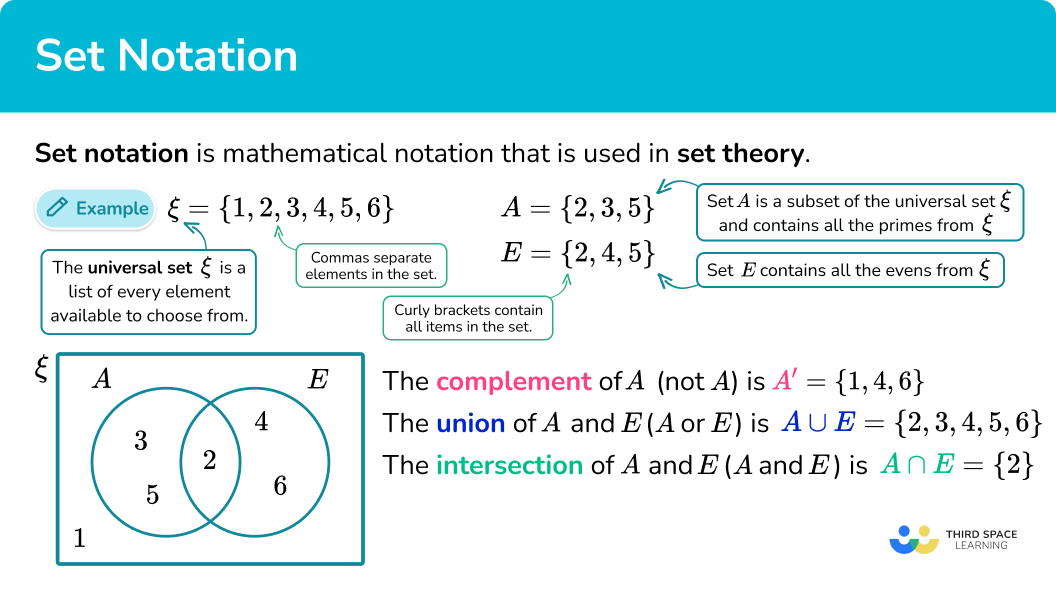## How to use set notation

In order to use set notation:

1. Define the elements in subsets of the universal set.
2. Decide what set notation is being used.
3. Write the required elements or values.

### How to use set notation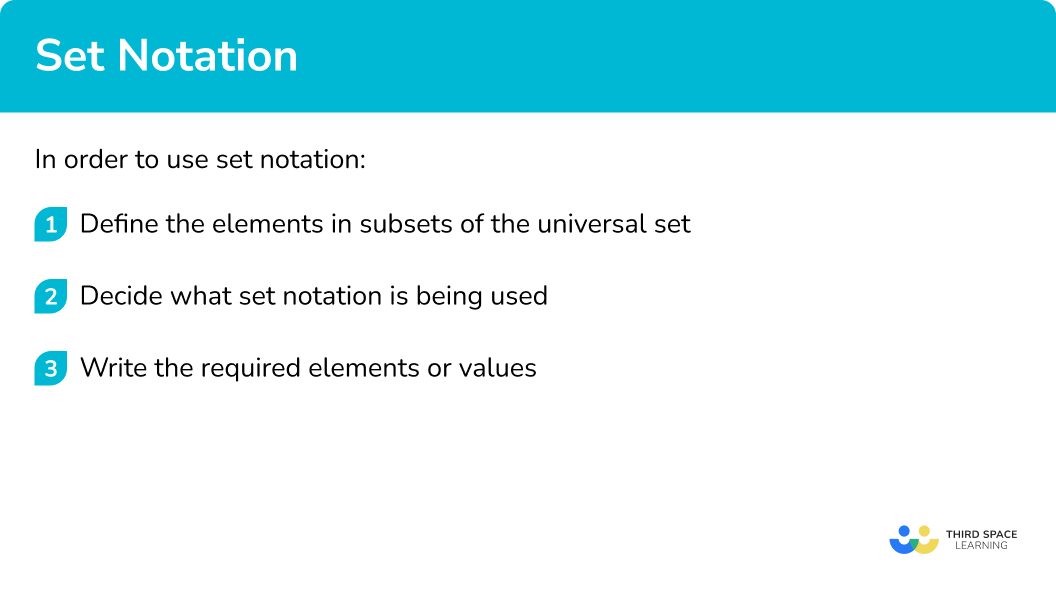## Related lessons on Venn diagram

Set notation is part of our series of lessons to support revision on how to calculate probability. You may find it helpful to start with the main Venn diagram lesson for a summary of what to expect, or use the step by step guides below for further detail on individual topics. Other lessons in this series include:

## Set notation examples

### Example 1: finding elements from listed sets

\xi = \{1, 2, 3, 4, 5, 6, 7, 8, 9, 10, 11, 12, 13, 14, 15\}

A = \{ multiples of 3\}

B = \{ factors of 12\}

Write down the elements in the set A \cup B .

1. Define the elements in subsets of the universal set.

A = \{ multiples of 3\} = \{3, 6, 9, 12, 15\}

B = \{ factors of 12\} = \{1, 2, 3, 4, 6, 12\}

2Decide what set notation is being used.

A \cup B means A union B .

We need to list the elements in set A or set B or both.

3Write the required elements or values.

A \cup B = \{1, 2, 3, 4, 6, 9, 12, 15\}

### Example 2: finding elements from listed sets

\xi = \{1, 2, 3, 4, 5, 6, 7, 8, 9, 10, 11, 12, 13, 14, 15\}

A = \{ multiples of 3 \}

B = \{ factors of 12 \}

Write down the elements in the set A \cap B.

A = \{ multiples of 3 \} = \{3, 6, 9, 12, 15\}

B = \{ factors of 12 \} = \{1, 2, 3, 4, 6, 12\}

A \cap B means A intersection B .

We need to list the elements in both set A and set B .

3, 6 and 12 are in both lists of elements.

A \cap B = \{3, 6, 12\}

### Example 3: finding elements from listed sets

\xi = \{ the integers 1 to 10\}

C = \{ the cube numbers \}

Write down the elements of the set C' .

\xi = \{1, 2, 3, 4, 5, 6, 7, 8, 9, 10\}

C = \{ the cube numbers \} = \{1, 8\}

C’ means the complement of C .

We need to list the elements not in set C .

C’ = \{2, 3, 4, 5, 6, 7, 9, 10\}

### Example 4: using set notation with a Venn diagram

For the Venn diagram below, list the elements in B’ .

\xi = \{ a, b, c, d, e, f, g, h, i, j \}

A = \{ a, b, c, d, e \}

B = \{ a, e, i \}

B’ means the complement of B .

We need to list the elements not in set B .

A’ = \{ b, c, d, f, g, h, j \}

### Example 5: using set notation with a Venn diagram

The Venn diagram shows the number of people attending classes at a gym with 50 members.

S = \{ spinning \}

P = \{ pilates \}

Find n ( S \cup P ).

S = \{ spinning \} had 22 attendees ( 15+7 ).

P = \{ pilates \} had 19 attendees ( 7+12 ).

n ( S \cup P ) means the cardinality of S union P .

We find the number of people who attended spinning or pilates or both.

S \cup P is the shaded region in this Venn diagram.

We need to add 15, 7 and 12 .

n ( S \cup P ) = 34

## Example 6: using set notation with a Venn diagram

The Venn diagram shows sets A and B .

Shade the region indicating ( A \cap B )’.

To define the sets A and B we can tick the regions in the Venn diagram.

Ticking the regions in set A gives,

Ticking the regions in set B gives,

A \cap B is the intersection of A and B . This is the region with both ticks, which is the middle region.

( A \cap B )’ is the complement of A \cap B . So we need everything apart from that middle region.

We can shade everything apart from the middle region.

## Example 7: using set notation with a Venn diagram

The Venn diagram shows sets A and B .

Shade the region indicating A’ \cup B .

To define the sets A’ and B we can tick the regions in the Venn diagram.

A’ means the complement of A so we can tick everything outside of set A .

Ticking the regions in set B gives,

A’ \cup B is the union of A’ and B . This is all of the regions with ticks.

We can shade the regions with ticks.

### Common misconceptions

• Confusing the union and intersection symbols
It is common to mix up the meanings of the symbols for union and intersection.
One way to help remember the meanings is that the \cap symbol will have the letter “ n ” in the word “and” and intersection. So A \cap B is A and B .

• Including elements more than once
A common error is to list the elements that are in both A and B twice when listing the elements or finding the cardinality for A \cup B .
If A =\{1, 2, 3, 4, 5, 6\} and B = \{4, 5, 6, 7, 8, 9\}, there are 6 elements in each set, however this does not mean that there are 12 elements in A \cup B .
A \cup B = \{1, 2, 3, 4, 5, 6, 7, 8, 9\} and has 9 elements.

• 1 is NOT prime
A common error with prime numbers is thinking that 1 is a prime number. 1 is NOT a prime number. Remember a prime number has exactly 2 factors, 1 and the number itself.

### Practice set notation questions

1) \xi = \{1, 2, 3, 4, 5, 6, 7, 8, 9, 10, 11, 12, 13, 14, 15, 16, 17, 18, 19, 20\}

A = \{ multiples of 4\}

Find n(A).

\{4, 8, 12, 16, 20\}\{1, 2, 4\}515n ( A ) means the cardinality of A , which is the number of elements in set A .

A = \{4, 8, 12, 16, 20\}, so there are 5 elements.

2) \xi = \{1, 2, 3, 4, 5, 6, 7, 8, 9, 10, 11, 12, 13, 14, 15, 16, 17, 18, 19, 20\}

A = \{ multiples of 4\}

B = \{ factors of 24\}

Write down the elements in A \cap B.

\{1, 2, 3, 4, 6, 8, 12, 16, 20, 24\}\{4, 8, 12\}310A = \{4, 8, 12, 16, 20\} and B = \{1, 2, 3, 4, 6, 8, 12, 24\}.

A \cap B is the intersection of A and B , so we need the elements that are in both A and B .

3) \xi = \{1, 2, 3, 4, 5, 6, 7, 8, 9, 10, 11, 12, 13, 14, 15, 16, 17, 18, 19, 20\}

C = \{ square numbers \}

D = \{even numbers \}

Write down the elements in C \cup D' .

\{1, 9\}\{4, 16\}\{1, 2, 4, 6, 8, 9, 10, 12, 14, 16, 18, 20\}\{1, 3, 4, 5, 7, 9, 11, 13, 15, 16, 17, 19\}C = \{1, 4, 9, 16\},D = \{2, 4, 6, 8, 10, 12, 14, 16, 18, 20\} and
D’ = \{1, 3, 5, 7, 9, 11, 13, 15, 17, 19\}.

C \cup D' means the union of C and D’ so it is the elements that appear in the lists of both C and D’ .

4) The Venn diagram shows the number of elements in subsets A and B of a universal set \xi .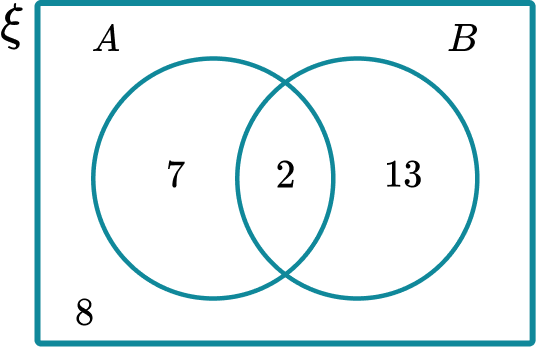Find n ( A' \cup B' ).

2283622A' \cup B' is the union of the complement of A and the complement of B . Tick the regions for A’ , tick the regions for B’ .

Shade all of the regions ticked and then add the values of the cardinality.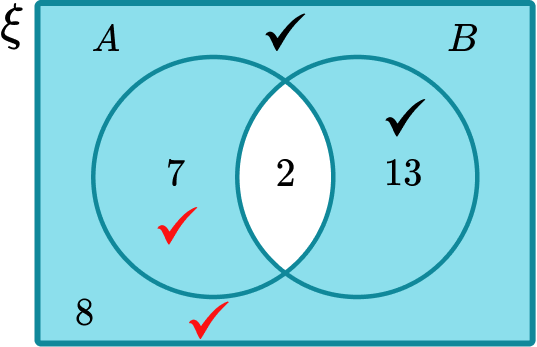5) The Venn diagram shows sets A and B .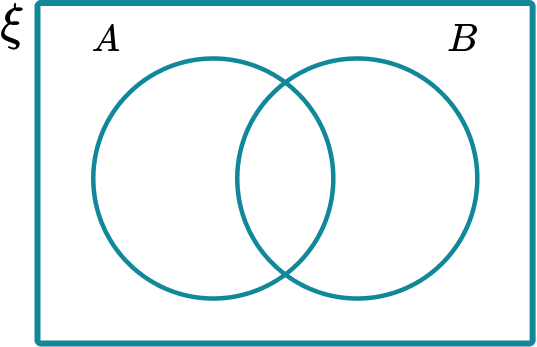Shade the region that satisfies A \cap B .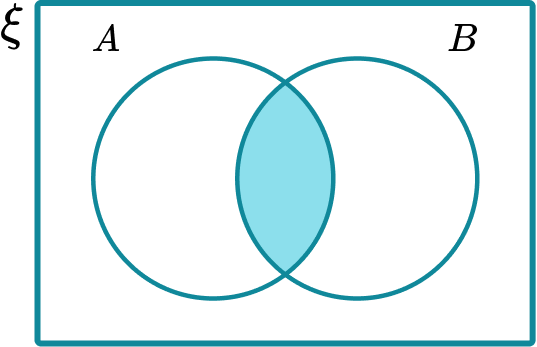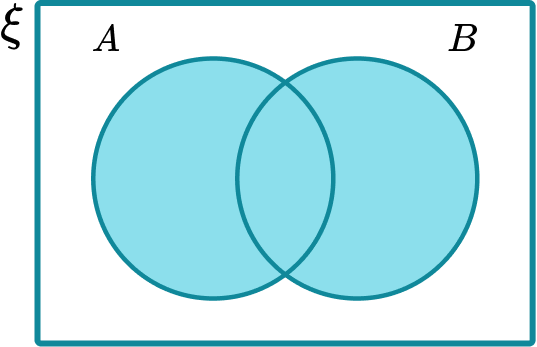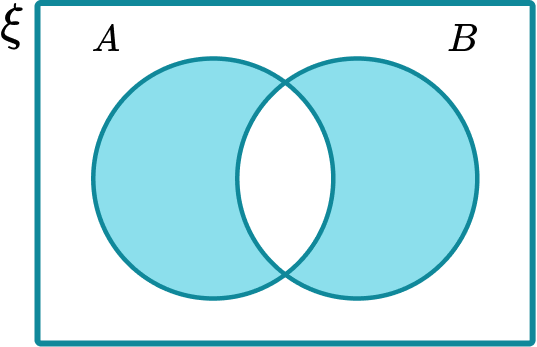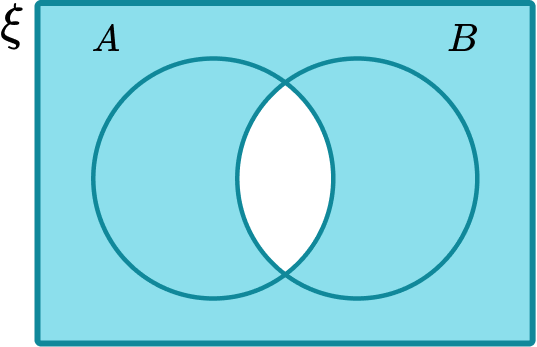A \cap B means the intersection of A and B . Tick the regions in A , then tick the regions in B . The region with the most ticks is the intersection.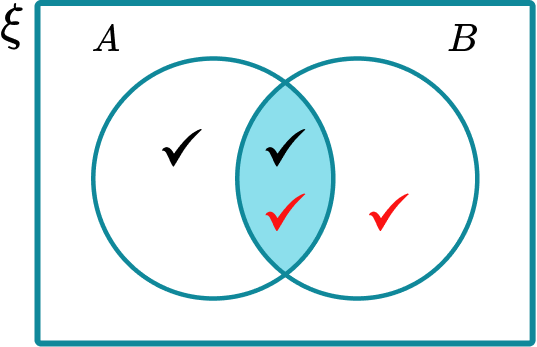6) What is the correct set notation for the shaded region shown in the Venn diagram?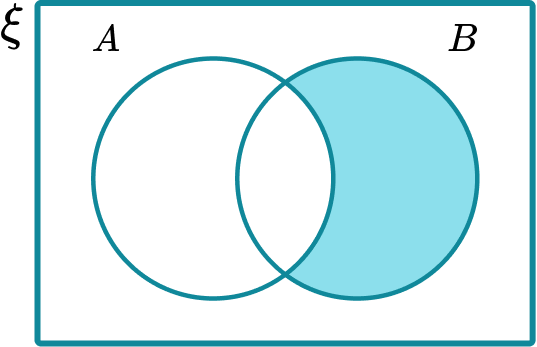A \cap B'A' \cup BA \cup B'A' \cap BA' \cap B means the intersection of A’ and B . Tick the regions in A’ , then tick the regions in B . The region with both ticks is the intersection.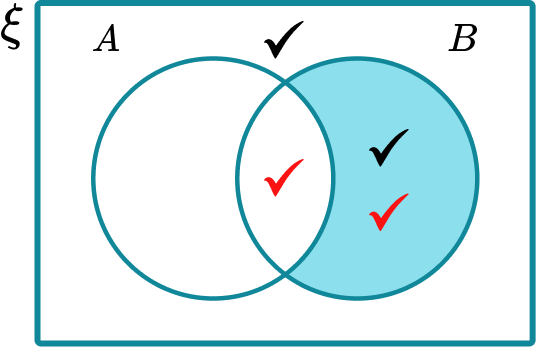### Set notation GCSE questions

1. Shade the region given by ( A \cup B )’.(2 marks)

The union of A and B indicated.

(1)

(1)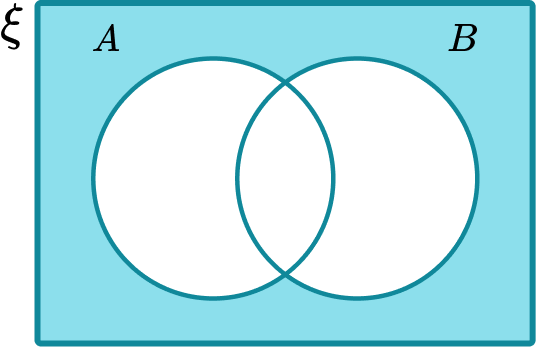2.  \xi = \{1, 2, 3, 4, 5, 6, 7, 8, 9, 10, 11, 12, 13, 14, 15\}

F = \{ factors of 36\}

M = \{ multiples of 3\}

(a) Write down the elements of F \cup M .

(2 marks)

(b) Write down the elements of F \cap M .

(1 mark)

(a) For lists of F or M \{1, 2, 3, 4, 6, 9, 12\} or \{3, 6, 9, 12, 15\}.

(1)

For correct list F \cup M 1, 2, 3, 4, 6, 9, 12, 15 .

(1)

(b) 3, 6, 9, 12

(1)

3. The Venn diagram shows elements in the universal set and subsets A and B .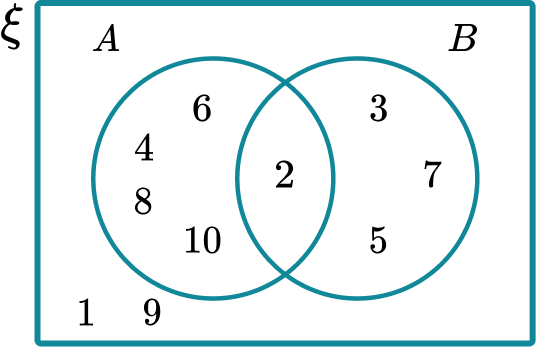(a) State what the set A represents.

(1 mark)

(b) State what the set B represents.

(1 mark)

(c) Write down the list of the numbers in A \cap B' .

(1 mark)

(a) Even numbers

(1)

(b) Prime numbers

(1)

(c) 4, 6, 8, 10

(1)

## Learning checklist

You have now learned how to:

• Use set notation to define sets, union of sets, intersection of set and compliments of sets

## Set notation in A level Mathematics

Set notation is not only used in probability. In A level mathematics, set notation is used to define solution sets to equations and inequalities.

For example,

The solutions to the quadratic equation x^2+2x-15=0 are x=-5 or x=3 .

Using set notation we would write this as \{x:x=-5\} \cup \{x:x=3\}.

We also use set notation to define different types of numbers.

The set of real numbers are denoted by

The set of integers are denoted by

The set of natural numbers are denoted by

The set of rational numbers are denoted by

## Still stuck?

Prepare your KS4 students for maths GCSEs success with Third Space Learning. Weekly online one to one GCSE maths revision lessons delivered by expert maths tutors.

Find out more about our GCSE maths tuition programme.